## Example Questions

← Previous 1 3 4 5 6 7 8 9 17 18

### Example Question #1 : How To Find A Fraction From A Percentage

Write as a fraction: 22%

11/50

2/3

11/100

4/9

4/7

11/50

Explanation:

22% = 22/100

Divide everything by 2:

22/100 = 11/50

11 is a prime number, so this is as reduced as this fraction can get.

### Example Question #2 : How To Find A Fraction From A Percentage

When y is decreased by ten percent, the result is equal to fifteen percent of x. Assuming both x and y are nonzero, what is the ratio of x to y?

6

1/6

1/3

3

18

6

Explanation:

The problem states that decreasing y by ten percent gives us the same thing as taking fifteen percent of x. We need to find an expression for decreasing y by ten percent, and an expression for fifteen percent of x, and then set these two things equal.

If we were to decrease y by ten percent, we would be left with ninety percent of y (because the percentages must add to one hundred percent). We could write ninety percent of y as 0.90y = (90/100)y = (9/10)y. Remember, when converting from a percent to a decimal, we need to move the decimal two places to the left.

Similarly, we can write 15% of x as 0.15x = (15/100)x = (3/20)x.

Now, we set these two expressions equal to one another.

(9/10)y = (3/20)x

Multiply both sides by 20 to eliminate fractions.

18y = 3x

The question asks us to find the ratio of x to y, which is equal to x/y. Thus, we must rearrange the equation above until we have x/y by itself on one side.

18y = 3x

Divide both sides by 3.

6y = x

Divide both sides by y.

6 = x/y

Thus, the ratio of x to y is 6.

### Example Question #1 : Fractions And Percentage

Write 7.5% as a fraction.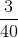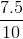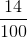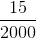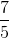Explanation:

First convert the percentage to a decimal:

7.5% = .075

Then turn this into a fraction:

.075 = 75/1000

Simplify by dividing the numerator and denominator by 25:

75/1000 = 3/40

### Example Question #4 : How To Find A Fraction From A Percentage

25% of 64 is equal to 5% of what number?

90

94

320

112

108

320

Explanation:

25% of 64 is 16 (you can find this with a calculator by 0.25 * 64). Divide 16 by 0.05 (or 1/20) to get the value of the number 16 is 5% of. (Or mental math of 16 * 20)

### Example Question #5 : How To Find A Fraction From A Percentage

Turn the following percentage into a fraction: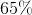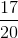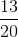Explanation:

Since a percentage is a "part of 100" to turn it into a fraction we take the original number in the percent and place it over 100:From here, simplify the fraction as necessary: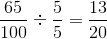### Example Question #6 : How To Find A Fraction From A Percentage

Turn the following percentage into a fraction: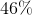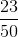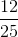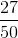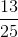Explanation:

Since a percentage is a "part of 100" to turn it into a fraction we take the original number in the percent and place it over 100: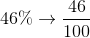From here, simplify the fraction as necessary: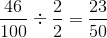### Example Question #7 : How To Find A Fraction From A Percentage

Turn the following percentage into a fraction: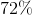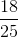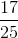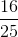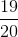Explanation:

Since a percentage is a "part of 100" to turn it into a fraction we take the original number in the percent and place it over 100: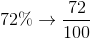From here, simplify the fraction as necessary: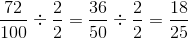### Example Question #8 : How To Find A Fraction From A Percentage

Turn the following percentage into a fraction: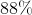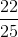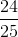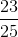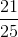Explanation:

Since a percentage is a "part of 100" to turn it into a fraction we take the original number in the percent and place it over 100: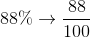From here, simplify the fraction as necessary: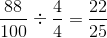### Example Question #9 : How To Find A Fraction From A Percentage

Turn the following percentage into a fraction: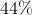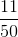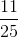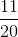Explanation:

Since a percentage is a "part of 100" to turn it into a fraction we take the original number in the percent and place it over 100: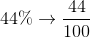From here, simplify the fraction as necessary: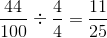### Example Question #10 : How To Find A Fraction From A Percentage

Turn the following percentage into a fraction: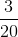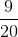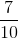Explanation:

Since a percentage is a "part of 100" to turn it into a fraction we take the original number in the percent and place it over 100: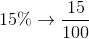From here, simplify the fraction as necessary: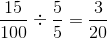← Previous 1 3 4 5 6 7 8 9 17 18